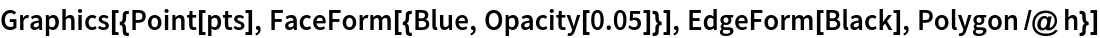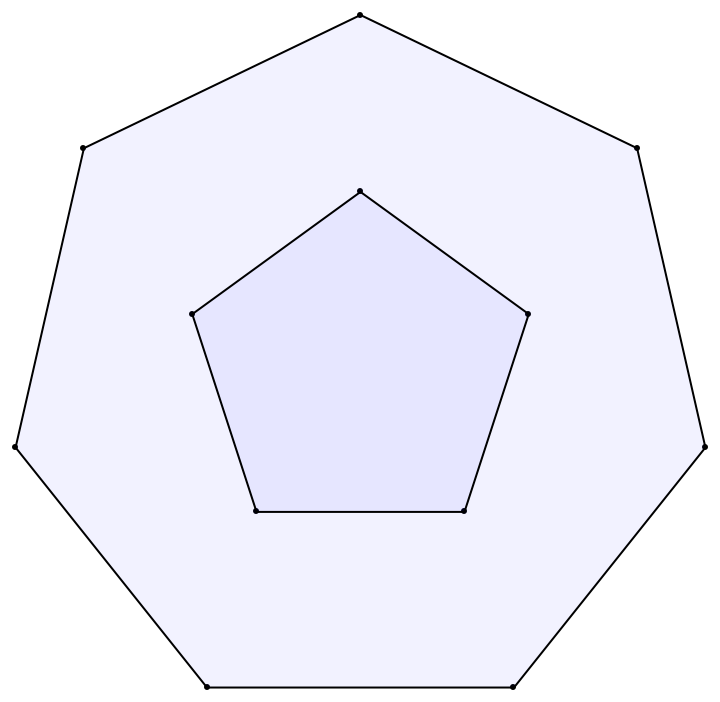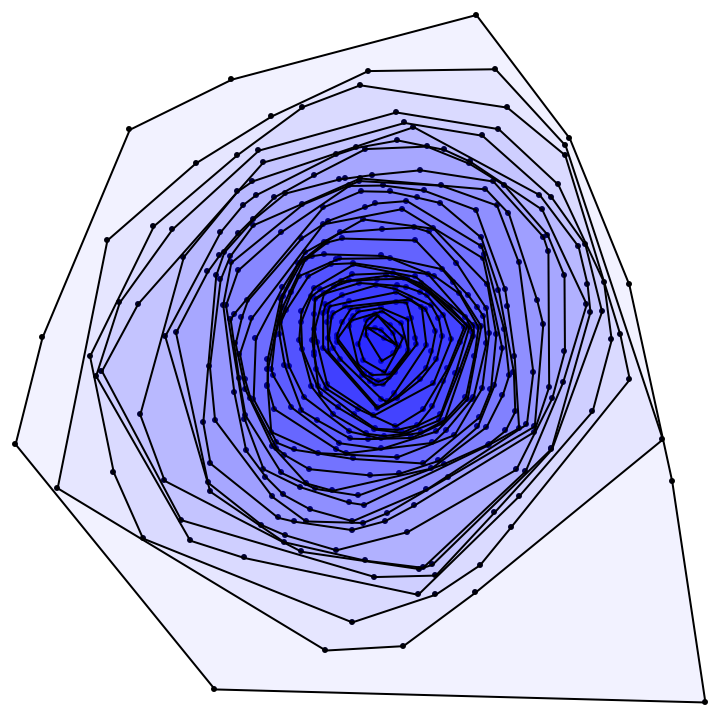Function Repository Resource:

# ConvexLayers2D

Calculate the convex layers of a set of 2D points

Contributed by: Sander Huisman
 ResourceFunction["ConvexLayers2D"][pts] calculates the convex layers for the points pts.

## Details

ResourceFunction["ConvexLayers2D"] returns a list of lists of points corresponding to each layer, starting with the outer layer and working inwards.
Each layer is a convex hull.

## Examples

### Basic Examples (2)

Define a list of points from a two polygons and then find the convex hull layers:

 In:=Out=Visualize the results:

 In:=Out=Calculate the convex layers for some randomly generated data:

 In:=Out=### Applications (1)

Visualize the layers:

 In:=Out=SHuisman

## Version History

• 1.0.0 – 02 September 2021